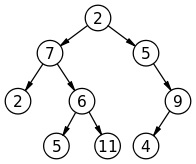Q:

# Print All Nodes that don't have Sibling

Given a Binary Tree write a program to print the nodes which don’t have a sibling node. Print all the nodes separated by space which don't have sibling in the tree in sorted order if every node has a tree than print -1.Explanation with example:

What is having sibling in tree?

A child node is said to have a sibling if the other node has the same parent as the child node. That means the nodes are siblings if they have same parent.

Like in the above example ‘2’ & ‘6’ are siblings as they have the same parent ‘7’.

In the above example the nodes that don’t have siblings are: 9, 4

Thus output:

4, 9 (sorted)

N.B: Root is not considered for sibling checking.

So, clearly a child node don’t have sibling, if its parent has only one pointer, either left or the right (that’s the child of course). Then we simply store the child node value & produce a sorted list to print. The traversal method we use here is level order traversal.

Algorithm:

```1. Define tree Node structure
2. FUNCTION printSibling (Node* root)
a) Declare a queue& a vector using STL
Queue<Node*>q;
vector<int>store;
b) push the root
EnQueue(q,root);
Node* temp;
While(queue is not empty) //do the level order traversal & check for siblings
temp=DeQueue(q);
//if the current node has only one child, definitely the child
//has no sibling, thus store the child node value
//left child pointer in NULL, right is not
If temp->left==NULL&&temp->right!=NULL
END IF
//right child pointer in NULL, left is not
If temp->left!=NULL&&temp->right==NULL
Add temp->left node value in store;
END IF
// do level order traversing
IF(temp->right)
EnQueue(q, temp->right);
IF(temp->left)
EnQueue(q, temp->left);
END WHILE
c) If no node found without having sibling then vector size is zero then print -1
d) Elsesort the vector to print sorted node value
END FUNCTION```

## C++ implementation to Print All Nodes that don't have Sibling

``````#include <bits/stdc++.h>
using namespace std;

// tree node is defined
class tree{
public:
int data;
tree *left;
tree *right;
};

void printSibling(tree* root)
{
//Declare queue using STL
queue<tree*> q;
//enqueue the root
q.push(root);
vector<int> store;

tree* temp;
//do the level order traversal & check for siblings
while(!q.empty()){
//dequeue
temp=q.front();
q.pop();
//if the current node has only one child
//definitely the child has no sibling
//store the child node value
if(temp->left==NULL && temp->right!=NULL){
store.push_back(temp->right->data);
}

if(temp->left!=NULL && temp->right==NULL){
store.push_back(temp->left->data);
}
// do level order traversing
if(temp->right)
q.push(temp->right);
if(temp->left)
q.push(temp->left);
}
//if no node found without having sibling
//vector size is zero
//print -1
if(store.size()==0){
printf("-1, no such  node\n");
return;
}
//sort the vector to print sorted node value
sort(store.begin(),store.end());
//printing
for(auto it=store.begin();it!=store.end();it++)
printf("%d ",*it);
}

tree* newnode(int data)  // creating new node
{
tree* node = (tree*)malloc(sizeof(tree));
node->data = data;
node->left = NULL;
node->right = NULL;

return(node);
}

int main()
{
//**same tree is builted as shown in example**
cout<<"same tree is built as shown in example\n";
tree *root=newnode(2);
root->left= newnode(7);
root->right= newnode(5);
root->right->right=newnode(9);
root->right->right->left=newnode(4);
root->left->left=newnode(2);
root->left->right=newnode(6);
root->left->right->left=newnode(5);
root->left->right->right=newnode(11);

cout<<"printing the nodes that don't have sibling...\n"<<endl;
printSibling(root);

return 0;
}
``````

Output

```same tree is built as shown in example
printing the nodes that don't have sibling...

4 9```